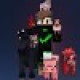# NCTB Class 6 Math Chapter One Exercise 1.4 Solution

NCTB Class 6 Math Chapter One Exercise 1.4 Solution by Math Expert. Bangladesh Board Class 6 Math Solution Chapter 1 Natural Numbers and Fractions Exercise 1.4 Solution.

 Board NCTB Class 6 Subject Mathematics Chapter 1 Chapter Name Natural Numbers and Fractions Exercise 1.4 Solution

### Exercise:1.4Updated: December 1, 2020 — 10:14 am

1.Nice thanks

1.2.3.I did not uderstand no.8

1.For fast solving you can use this method Imtiaz

4.7b was 9-3 15/16 not 9-3 15/65

5.7b ans is 3 3/32

6.where is 11 number math